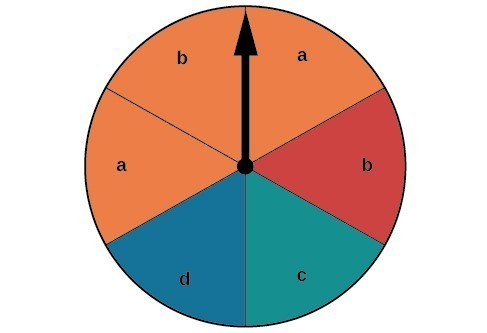## Computing the Probability of the Union of Two Events

We are often interested in finding the probability that one of multiple events occurs. Suppose we are playing a card game, and we will win if the next card drawn is either a heart or a king. We would be interested in finding the probability of the next card being a heart or a king. The union of two events $E\text{ and }F,\text{written }E\cup F$, is the event that occurs if either or both events occur.

$P\left(E\cup F\right)=P\left(E\right)+P\left(F\right)-P\left(E\cap F\right)$

Suppose the spinner in Figure 2 is spun. We want to find the probability of spinning orange or spinning a $b$.Figure 2

There are a total of 6 sections, and 3 of them are orange. So the probability of spinning orange is $\frac{3}{6}=\frac{1}{2}$. There are a total of 6 sections, and 2 of them have a $b$. So the probability of spinning a $b$ is $\frac{2}{6}=\frac{1}{3}$. If we added these two probabilities, we would be counting the sector that is both orange and a $b$ twice. To find the probability of spinning an orange or a $b$, we need to subtract the probability that the sector is both orange and has a $b$.

$\frac{1}{2}+\frac{1}{3}-\frac{1}{6}=\frac{2}{3}$

The probability of spinning orange or a $b$ is $\frac{2}{3}$.

### A General Note: Probability of the Union of Two Events

The probability of the union of two events $E$ and $F$ (written $E\cup F$ ) equals the sum of the probability of $E$ and the probability of $F$ minus the probability of $E$ and $F$ occurring together $\text{(}$ which is called the intersection of $E$ and $F$ and is written as $E\cap F$ ).

$P\left(E\cup F\right)=P\left(E\right)+P\left(F\right)-P\left(E\cap F\right)$

### Example 3: Computing the Probability of the Union of Two Events

A card is drawn from a standard deck. Find the probability of drawing a heart or a 7.

### Solution

A standard deck contains an equal number of hearts, diamonds, clubs, and spades. So the probability of drawing a heart is $\frac{1}{4}$. There are four 7s in a standard deck, and there are a total of 52 cards. So the probability of drawing a 7 is $\frac{1}{13}$.

The only card in the deck that is both a heart and a 7 is the 7 of hearts, so the probability of drawing both a heart and a 7 is $\frac{1}{52}$. Substitute $P\left(H\right)=\frac{1}{4}, P\left(7\right)=\frac{1}{13}, \text{and} P\left(H\cap 7\right)=\frac{1}{52}$ into the formula.

$\begin{array}{l}P\left(E{\cup }^{\text{ }}F\right)=P\left(E\right)+P\left(F\right)-P\left(E{\cap }^{\text{ }}F\right)\hfill \\ \text{ }=\frac{1}{4}+\frac{1}{13}-\frac{1}{52}\hfill \\ \text{ }=\frac{4}{13}\hfill \end{array}$

The probability of drawing a heart or a 7 is $\frac{4}{13}$.

### Try It 3

A card is drawn from a standard deck. Find the probability of drawing a red card or an ace.

Solution